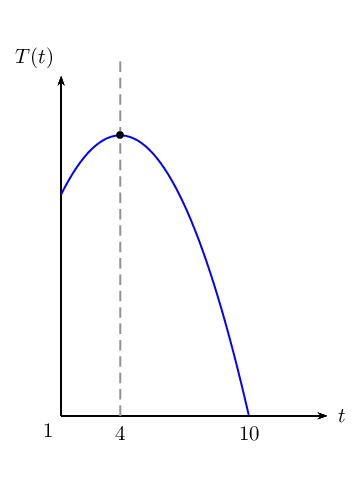Home Practice
For learners and parents For teachers and schools
Textbooks
Full catalogue
Pricing SupportLog in

We think you are located in United States. Is this correct?

# 6.7 Applications of differential calculus

## 6.7 Applications of differential calculus (EMCHH)

temp text

### Optimisation problems (EMCHJ)

We have seen that differential calculus can be used to determine the stationary points of functions, in order to sketch their graphs. Calculating stationary points also lends itself to the solving of problems that require some variable to be maximised or minimised. These are referred to as optimisation problems.

The fuel used by a car is defined by $$f\left(v\right)=\frac{3}{80}{v}^{2}-6v+245$$, where $$v$$ is the travelling speed in $$\text{km/h}$$.

What is the most economical speed of the car? In other words, determine the speed of the car which uses the least amount of fuel.

If we draw the graph of this function we find that the graph has a minimum. The speed at the minimum would then give the most economical speed.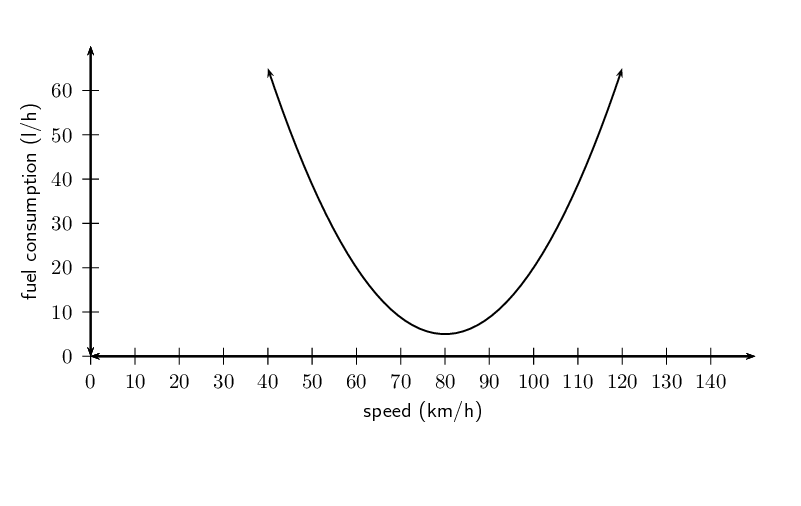We have seen that the coordinates of the turning point can be calculated by differentiating the function and finding the $$x$$-coordinate (speed in the case of the example) for which the derivative is $$\text{0}$$.

${f}'\left(v\right)=\frac{3}{40}v-6$

If we set $${f}'\left(v\right)=0$$ we can calculate the speed that corresponds to the turning point:

\begin{align*} {f}'\left(v\right) & = \frac{3}{40}v-6 \\ 0 & = \frac{3}{40}v-6 \\ v & = \frac{6\times 40}{3} \\ & = 80 \end{align*}

This means that the most economical speed is $$\text{80}\text{ km/h}$$.

Finding the optimum point:

Let $$f'(x) = 0$$ and solve for $$x$$ to find the optimum point.

To check whether the optimum point at $$x = a$$ is a local minimum or a local maximum, we find $$f''(x)$$:

• If $$f''(a) < 0$$, then the point is a local maximum.

• If $$f''(a) > 0$$, then the point is a local minimum.

## Worked example 21: Optimisation problems

The sum of two positive numbers is $$\text{10}$$. One of the numbers is multiplied by the square of the other. If each number is greater than $$\text{0}$$, find the numbers that make this product a maximum.

Draw a graph to illustrate the answer.

### Analyse the problem and formulate the equations that are required

Let the two numbers be $$a$$ and $$b$$ and the product be $$P$$.

\begin{align*} a+b &=10 \ldots \ldots (1) \\ P &= a \times b^{2} \ldots \ldots (2) \end{align*}

Make $$b$$ the subject of equation ($$\text{1}$$) and substitute into equation ($$\text{2}$$):

\begin{align*} P &= a\left(10-a\right)^{2} \\ &=a \left( 100 - 20a + a^{2} \right) \\ \therefore P(a) &= 100a - 20a^{2} + a^{3} \end{align*}

### Differentiate with respect to $$a$$

${P}'\left(a\right)= 100 - 40a + 3a^{2}$

### Determine the stationary points by letting $$P'(a) = 0$$

We find the value of $$a$$ which makes $$P$$ a maximum:

\begin{align*} {P}'\left(a\right) & = 3a^{2} -40a + 100 \\ 0 &= (3a - 10)(a - 10) \\ \therefore a = 10 & \text{ or } a = \frac{10}{3} \end{align*}

Substitute into the equation ($$\text{1}$$) to solve for $$b$$:

\begin{align*} \text{If } a = 10 : \enspace b & = 10 - 10 \\ & = 0 \enspace (\text{but } b > 0 ) \\ \therefore \text{no solution} \\ \\ \text{If } a = \frac{10}{3} : \enspace b & = 10 - \frac{10}{3} \\ & = \frac{20}{3} \end{align*}

### Determine the second derivative $$P''(a)$$

We check that the point $$\left(\frac{10}{3};\frac{20}{3}\right)$$ is a local maximum by showing that $${P}''\left(\frac{10}{3}\right) < 0$$:

\begin{align*} {P}''\left(a\right) & = 6a -40 \\ \therefore {P}''\left(\frac{10}{3}\right) & = 6\left(\frac{10}{3}\right) -40 \\ & = 20 -40 \\ & = -20 \end{align*}

The product is maximised when the two numbers are $$\frac{10}{3}$$ and $$\frac{20}{3}$$.

### Draw a graph

To draw a rough sketch of the graph we need to calculate where the graph intersects with the axes and the maximum and minimum function values of the turning points:

Intercepts:

\begin{align*} P(a) &= a^{3} -20a^{2} + 100a \\ &= a(a - 10)^{2} \\ & \\ \text{Let } P(a) = 0: \enspace & (0;0) \text{ and } (10;0) \end{align*}

Turning points:

\begin{align*} P'(a) & = 0 \\ \therefore a = \frac{10}{3} & \text{ or } a = 10 \end{align*}

Maximum and minimum function values:

\begin{align*} \text{Substitute } \left( \frac{10}{3}; \frac{20}{3} \right): \enspace P &= ab^{2} \\ &= \left( \frac{10}{3} \right) \left( \frac{20}{3} \right)^{2} \\ &= \frac{4000}{27} \\ & \approx 148 \qquad (\text{Maximum turning point})\\ & \\ \text{Substitute } (0;10): \enspace P &= ab^{2} \\ &= \left( 10 \right) \left( 0 \right)^{2} \\ &= 0 \qquad (\text{Minimum turning point}) \end{align*}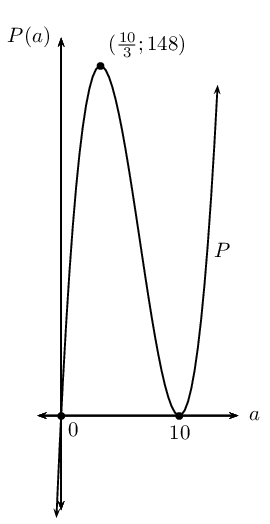Note: the above diagram is not drawn to scale.

## Worked example 22: Optimisation problems

Michael wants to start a vegetable garden, which he decides to fence off in the shape of a rectangle from the rest of the garden. Michael has only $$\text{160}\text{ m}$$ of fencing, so he decides to use a wall as one border of the vegetable garden. Calculate the width and length of the garden that corresponds to the largest possible area that Michael can fence off.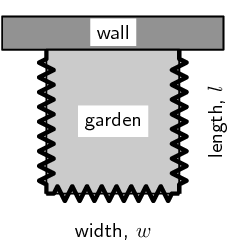### Examine the problem and formulate the equations that are required

The important pieces of information given are related to the area and modified perimeter of the garden. We know that the area of the garden is given by the formula:

$\text{Area }= w \times l$

The fencing is only required for $$\text{3}$$ sides and the three sides must add up to $$\text{160}\text{ m}$$.

$160 = w + l + l$

Rearrange the formula to make $$w$$ the subject of the formula:

$w=160 -2 l$

Substitute the expression for $$w$$ into the formula for the area of the garden. Notice that this formula now contains only one unknown variable.

\begin{align*} \text{Area } &= l(160 - 2l) \\ &= 160l - 2l^{2} \end{align*}

### Differentiate with respect to $$l$$

We are interested in maximising the area of the garden, so we differentiate to get the following:

\begin{align*} \frac{dA}{dl} = A' &= 160 - 4l \end{align*}

### Calculate the stationary point

To find the stationary point, we set $${A}'\left(l\right)=0$$ and solve for the value(s) of $$l$$ that maximises the area:

\begin{align*} {A}'\left(l\right) & = 160 - 4l \\ 0 &= 160 - 4l \\ 4l &= 160 \\ \therefore l &= 40 \end{align*}

Therefore, the length of the garden is $$\text{40}\text{ m}$$.

Substitute to solve for the width:

\begin{align*} w & = 160 -2l \\ & = 160 -2\left(40 \right) \\ & = 160 - 80 \\ & = 80 \end{align*}

Therefore, the width of the garden is $$\text{80}\text{ m}$$.

### Determine the second derivative $${A}''\left(l\right)$$

We can check that this gives a maximum area by showing that $${A}''\left(l\right) < 0$$:

${A}''\left(l\right) = -4$

A width of $$\text{80}\text{ m}$$ and a length of $$\text{40}\text{ m}$$ will give the maximum area for the garden.

Important note:

The quantity that is to be minimised or maximised must be expressed in terms of only one variable. To find the optimised solution we need to determine the derivative and we only know how to differentiate with respect to one variable (more complex rules for differentiation are studied at university level).

## Solving optimisation problems

Textbook Exercise 6.11

The sum of two positive numbers is $$\text{20}$$. One of the numbers is multiplied by the square of the other. Find the numbers that make this product a maximum.

Let the first number be $$x$$ and the second number be $$y$$ and let the product be $$P$$. We get the following two equations:

\begin{align*} x + y & = 20 \\ xy^{2} & = P \end{align*}

Rearranging the first equation and substituting into the second gives:

\begin{align*} P & = (20 - x)^{2}x \\ & = 400x - 40x^{2} + x^{3} \end{align*}

Differentiating and setting to $$\text{0}$$ gives:

\begin{align*} P' & = 400 - 80x + 3x^{2} \\ 0 & = 3x^{2} - 80x + 400 \\ & = (3x-20)(x-20) \end{align*}

Therefore, $$x=20$$ or $$x=\frac{20}{3}$$.

If $$x=20$$ then $$y=0$$ and the product is a minimum, not a maximum.

Therefore, $$x=\frac{20}{3}$$ and $$y=20-\frac{20}{3} = \frac{40}{3}$$.

Therefore the two numbers are $$\frac{20}{3}$$ and $$\frac{40}{3}$$ (approximating to the nearest integer gives $$\text{7}$$ and $$\text{13}$$).

A wooden block is made as shown in the diagram. The ends are right-angled triangles having sides $$3x$$, $$4x$$ and $$5x$$. The length of the block is $$y$$. The total surface area of the block is $$\text{3 600}\text{ cm^{2}}$$.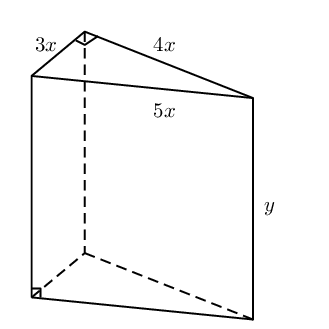Show that $$y= \frac{\text{300} - x^{2}}{x}$$.

We start by finding the surface area of the prism:

\begin{align*} \text{Surface area} & = 2 \left(\frac{1}{2}b \times h\right) + 3xy + 4xy +5xy \\ \text{3 600} & = (3x \times 4x) + 12xy \\ & = 12x^{2} + 12 xy \end{align*}

Solving for $$y$$ gives:

\begin{align*} 12 xy & = \text{3 600} - 12x^{2} \\ y & = \frac{\text{3 600} - 12x^{2}}{12 x}\\ y& = \frac{\text{300} - x^{2}}{x} \end{align*}

Find the value of $$x$$ for which the block will have a maximum volume.

(Volume = area of base $$\times$$ height)

Start by finding an expression for volume in terms of $$x$$:

\begin{align*} V&=\text{ area of triangle } \times y \\ V & = 6x^{2} \times \frac{\text{300} - x^{2}}{x} \\ & = 6x ( \text{300} - x^{2})\\ & = \text{1 800}x - 6x^{3} \end{align*}

Now take the derivative and set it equal to $$\text{0}$$:

\begin{align*} V' & = \text{1 800} - 18x^{2} \\ 0 & = \text{1 800} - 18x^{2} \\ 18x^{2} & = \text{1 800} \\ x^{2} & = 100\\ x & = \pm 10 \end{align*}

Since the length can only be positive, $$x=10$$

$$\therefore x = \text{10}\text{ cm}$$

Determine the shortest vertical distance between the curves of $$f$$ and $$g$$ if it is given that: \begin{align*} f(x)&= -x^{2}+2x+3 \\ \text{and } g(x)&= \frac{8}{x}, \quad x > 0 \end{align*}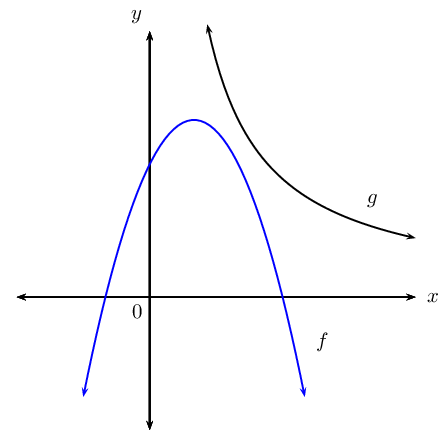\begin{align*} \text{Let the distance } P(x) &= g(x) - f(x)\\ &=\frac{8}{x} - (-x^{2}+2x+3) \\ &=\frac{8}{x} +x^{2} - 2x - 3 \end{align*}

To minimise the distance between the curves, let $$P'(x) = 0:$$

\begin{align*} P'(x) &= - \frac{8}{x^2 } + 2x - 2 \qquad (x \ne 0) \\ 0 &= - \frac{8}{x^2 } + 2x - 2 \\ \therefore 0 &= - 8 + 2x^3 - 2x \\ 0 &= 2x^3 - 2x - 8 \\ 0 &= x^3 - x - 4 \\ 0 &= (x - 2)(x^2 + x + 2) \\ \therefore x =2 & \text{ or } x = \frac{-1 \pm \sqrt{(1)^2 - 4(1)(2)}}{2(1)} \\ & \quad = \text{ no real solutions } \\ \therefore x & =2 \end{align*}

Therefore, the shortest distance:

\begin{align*} P(2) &=\frac{8}{(2)} +(2)^{2} - 2(2) - 3 \\ &= 4 + 4 - 4 -3 \\ &= \text{1}\text{ unit} \end{align*}

The diagram shows the plan for a verandah which is to be built on the corner of a cottage. A railing $$ABCDE$$ is to be constructed around the four edges of the verandah.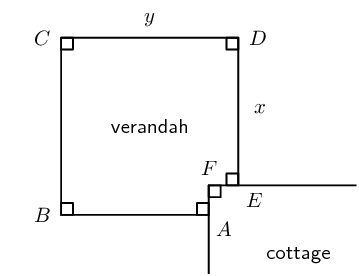If $$AB=DE=x$$ and $$BC=CD=y$$, and the length of the railing must be $$\text{30}\text{ m}$$, find the values of $$x$$ and $$y$$ for which the verandah will have a maximum area.

We need to determine an expression for the area in terms of only one variable.

The perimeter is:

\begin{align*} P & = 2x + 2y \\ 30 & = 2x + 2y \\ 15 & = x + y \\ y&=15-x \end{align*}

The area is:

\begin{align*} A & = y^{2} - (y-x)^{2} \\ & = y^{2} - (y^{2} - 2xy + x^{2}) \\ & = y^{2} - y^{2} + 2xy - x^{2} \\ & = 2xy - x^{2} \end{align*}

We use the expression for perimeter to eliminate the $$y$$ variable so that we have an expression for area in terms of $$x$$ only:

\begin{align*} A(x) & = 2x(15-x) - x^{2} \\ & = 30x - 2x^{2} - x^{2} \\ & = 30x - 3x^{2} \end{align*}

To find the maximum, we need to take the derivative and set it equal to $$\text{0}$$:

\begin{align*} A'(x) & = 30 - 6x \\ 0 & = 30 - 6x \\ 6x & = 30 \\ x & = 5 \end{align*}

Therefore, $$x=\text{5}\text{ m}$$ and substituting this value back into the formula for perimeter gives $$y=\text{10}\text{ m}$$.

A rectangular juice container, made from cardboard, has a square base and holds $$\text{750}\text{ cm}^{3}$$ of juice. The container has a specially designed top that folds to close the container. The cardboard needed to fold the top of the container is twice the cardboard needed for the base, which only needs a single layer of cardboard.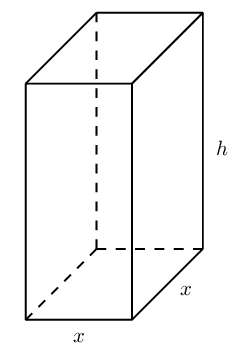If the length of the sides of the base is $$x$$ cm, show that the total area of the cardboard needed for one container is given by: $A (\text{in square centimetres}) = \frac{\text{3 000}}{x} + 3x^{2}$

\begin{align*} V & = x^2h \\ 750 & = x^2h \\ \therefore h & = \frac{750}{x^2}\\ A & = \text{ area of sides } + \text{ area of base } + \text{ area of top } \\ &= 4xh + x^2 + 2x^2 \\ &= 4xh + 3x^2 \\ \text{Substitute } h &= \frac{750}{x^2}: \\ A &= 4x\left( \frac{750}{x^2} \right) + 3x^2 \\ &= \frac{3000}{x}+ 3x^2 \end{align*}

Determine the dimensions of the container so that the area of the cardboard used is minimised.

\begin{align*} A(x) &= \frac{3000}{x}+ 3x^2 \\ A'(x) &= - \frac{3000}{x^2}+ 6x \\ \therefore 0 &= - \frac{3000}{x^2}+ 6x \\ 6x &= \frac{3000}{x^2} \\ x^3 &= 500 \\ \therefore x &= \sqrt{500} \\ &\approx \text{7,9}\text{ cm} \\ \therefore h & = \frac{750}{(\text{7,9})^2}\\ &\approx \text{12,0}\text{ cm} \end{align*}

### Rates of change (EMCHK)

temp text

It is very useful to determine how fast (the rate at which) things are changing. Mathematically we can represent change in different ways. For example we can use algebraic formulae or graphs.

Graphs give a visual representation of the rate at which the function values change as the independent (input) variable changes. This rate of change is described by the gradient of the graph and can therefore be determined by calculating the derivative.

We have learnt how to determine the average gradient of a curve and how to determine the gradient of a curve at a given point. These concepts are also referred to as the average rate of change and the instantaneous rate of change.

$\text{Average rate of change } = \frac{f\left(x+h\right)-f\left(x\right)}{(x + h) - x}$ $\text{Instantaneous rate of change } = \lim_{h\to 0}\frac{f\left(x+h\right)-f\left(x\right)}{h}$

When we mention rate of change, the instantaneous rate of change (the derivative) is implied. When average rate of change is required, it will be specifically referred to as average rate of change.

Velocity is one of the most common forms of rate of change:

\begin{align*} \text{Average velocity } &= \text{Average rate of change } \\ & \\ \text{Instantaneous velocity } &= \text{Instantaneous rate of change } \\ &= \text{Derivative} \end{align*}

Velocity refers to the change in distance ($$s$$) for a corresponding change in time ($$t$$).

$v\left(t\right)=\frac{ds}{dt}={s}'\left(t\right)$

Acceleration is the change in velocity for a corresponding change in time. Therefore, acceleration is the derivative of velocity

$a\left(t\right)={v}'\left(t\right)$

This implies that acceleration is the second derivative of the distance.

$a\left(t\right)={s}''\left(t\right)$

## Worked example 23: Rate of change

The height (in metres) of a golf ball $$t$$ seconds after it has been hit into the air, is given by $$H\left(t\right)=20t-5{t}^{2}$$. Determine the following:

1. The average vertical velocity of the ball during the first two seconds.

2. The vertical velocity of the ball after $$\text{1,5}$$ $$\text{s}$$.

3. The time at which the vertical velocity is zero.

4. The vertical velocity with which the ball hits the ground.

5. The acceleration of the ball.

### Determine the average vertical velocity during the first two seconds

\begin{align*} {v}_{\text{ave}} & = \frac{H\left(2\right)-H\left(0\right)}{2-0} \\ & = \frac{\left[20\left(2\right)-5{\left(2\right)}^{2}\right]-\left[20\left(0\right)-5{\left(0\right)}^{2}\right]}{2} \\ & = \frac{40-20}{2} \\ & = \text{10}\text{ m.s$^{-1}$}\end{align*}

### Calculate the instantaneous vertical velocity

\begin{align*} v\left(t\right) & = H'(t) \\ &= \frac{dH}{dt} \\ & = 20-10t \end{align*}

Velocity after $$\text{1,5}$$ $$\text{s}$$:

\begin{align*} v\left(\text{1,5}\right) & = 20-10\left(\text{1,5}\right) \\ & = \text{5}\text{ m.s$^{-1}$} \end{align*}

### Determine the time at which the vertical velocity is zero

\begin{align*} v\left(t\right) & = 0 \\ 20-10t & = 0 \\ 10t & = 20 \\ t & = 2 \end{align*}

Therefore, the velocity is zero after $$\text{2}\text{ s}$$

### Find the vertical velocity with which the ball hits the ground

The ball hits the ground when $$H\left(t\right)=0$$

\begin{align*} 20t-5{t}^{2} & = 0 \\ 5t\left(4-t\right) & = 0 \\ t=0& \text{ or } t=4 \end{align*}

The ball hits the ground after $$\text{4}$$ $$\text{s}$$. The velocity after $$\text{4}$$ $$\text{s}$$ will be:

\begin{align*} v\left(4\right) & = {H}'\left(4\right) \\ & = 20-10\left(4\right) \\ & = -\text{20}\text{ m.s$^{-1}$} \end{align*}

The ball hits the ground at a speed of $$\text{20}\text{ m.s^{-1}}$$. Notice that the sign of the velocity is negative which means that the ball is moving downward (a positive velocity is used for upwards motion).

### Acceleration

\begin{align*} a=v'(t)&=H''(t) \\ &=-10 \end{align*}\begin{align*} \therefore a & = -\text{10}\text{ m.s$^{-2}$} \end{align*}

Just because gravity is constant does not mean we should necessarily think of acceleration as a constant. We should still consider it a function.

## Rates of change

Textbook Exercise 6.12

A pump is connected to a water reservoir. The volume of the water is controlled by the pump and is given by the formula: \begin{align*} V(d)&=64+44d-3d^{2} \\ \text{where } V&= \text{ volume in kilolitres}\\ d&= \text{ days} \end{align*}

Determine the rate of change of the volume of the reservoir with respect to time after $$\text{8}$$ days.
\begin{align*} \text{Rate of change }&= V'(d) \\ V'(d)&= 44 -6d \\ \text{After 8 days, rate of change will be:}\\ V'(8)&=44-6(8)\\ &= -\text{4}\text{ kℓ per day} \end{align*}
Is the volume of the water increasing or decreasing at the end of $$\text{8}$$ days. Explain your answer.

The rate of change is negative, so the function is decreasing.

After how many days will the reservoir be empty?
\begin{align*} \text{Reservoir empty: } V(d)&=0 \\ \therefore 64 + 44d -3d^{2}&=0 \\ (16-d)(4+3d)&=0\\ \therefore d = 16 \text{ or } & d = -\frac{4}{3} \\ \therefore \text{ It will be empty after } \text{16}\text{ days} \end{align*}
When will the amount of water be at a maximum?

Maximum at turning point.

\begin{align*} \text{Turning point where } V'(d)&= 0 \\ \therefore 44 - 6d&=0\\ d &=\frac{44}{6} \\ &= 7\frac{1}{3} \text{ days} \end{align*}
Calculate the maximum volume.

Maximum at turning point.

\begin{align*} \text{Maximum volume } &= V\left(\frac{22}{3}\right) \\ V\left(\frac{22}{3}\right) &= 64 + 44\left(\frac{22}{3}\right) - 3\left(\frac{22}{3}\right)^{2} \\ &= \text{225,3}\text{ kℓ} \end{align*}
Draw a graph of $$V(d)$$.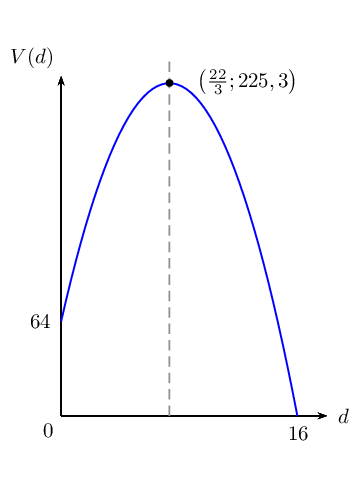A soccer ball is kicked vertically into the air and its motion is represented by the equation: \begin{align*} D(t)&=1 + 18t -3t^{2} \\ \text{where } D &= \text{distance above the ground (in metres)} \\ t&= \text{ time elapsed (in seconds)} \end{align*}

Determine the initial height of the ball at the moment it is being kicked.
\begin{align*} D(t)&=1 + 18t - 3t^{2} \\ D(0)&=1 + 18(0) - 3(0)^{2} \\ &= 1 \text{ metre} \end{align*}
Find the initial velocity of the ball.
\begin{align*} \text{Velocity } = D'(t) &= 18 - 6t \\ \text{Initial velocity } &= D'(0) \\ D'(0) =18 - 6(0) &=\text{18}\text{ m.s$^{-1}$} \end{align*}
Determine the velocity of the ball after $$\text{1,5}$$ $$\text{s}$$.
\begin{align*} \text{Velocity after } \text{1,5}\text{ s}&=D'(\text{1,5}) \\ D'(\text{1,5})&=18-6(\text{1,5})^{2} \\ &=18-9 \\ &=\text{9}\text{ m.s$^{-1}$} \end{align*}
Calculate the maximum height of the ball.

Maximum height is at the turning point.

\begin{align*} \text{Turning point: } D'(t)&=0 \\ \therefore 18 - 6t&=0\\ 6t&=18 \\ t&=\text{3}\text{ s} \\ \text{Maximum height }&=D(3) \\ &=1+(18)(3)-(3)(3)^{2} \\ &=\text{28}\text{ metres} \end{align*}
Determine the acceleration of the ball after $$\text{1}$$ second and explain the meaning of the answer.
\begin{align*} \text{Acceleration }&= D''(t) \\ D''(t)&= -\text{6}\text{ m.s$^{-2}$} \end{align*}

Interpretation: the velocity is decreasing by $$\text{6}$$ metres per second per second.

Calculate the average velocity of the ball during the third second.

Average velocity during third second:

\begin{align*} &= \frac{D(3)-D(2)}{3-2} \\ &= \frac{1+18(3)-3(3)^{2}-\left[1+18(2)-3(2)^{2}\right]}{1} \\ &= \text{3}\text{ m.s$^{-1}$} \end{align*}
Determine the velocity of the ball after $$\text{3}$$ seconds and interpret the answer.
\begin{align*} \text{Instantaneous velocity}&= D'(3) \\ &= 18-6(3) \\ &= \text{0}\text{ m.s$^{-1}$} \end{align*}

Interpretation: this is the stationary point, where the derivative is zero. The ball has stopped going up and is about to begin its descent.

How long will it take for the ball to hit the ground?
\begin{align*} \text{Hits ground: } D(t)&=0 \\ -3t^{2}+18t+1&=0\\ t&=\frac{-18 \pm\sqrt{(18^{2}-4(1)(-3)}}{2(-3)} \\ t&=\frac{-18\pm\sqrt{336}}{-6} \\ \therefore t&=-\text{0,05} \text{ or } t=\text{6,05} \end{align*}

The ball hits the ground at $$\text{6,05}$$ $$\text{s}$$ (time cannot be negative).

Determine the velocity of the ball when it hits the ground.
\begin{align*} \text{Velocity after } \text{6,05}\text{ s}&= D'(\text{6,05}) \\ D'(\text{6,05})=18-5(\text{6,05})&= -\text{18,3}\text{ m.s$^{-1}$} \end{align*}

If the displacement $$s$$ (in metres) of a particle at time $$t$$ (in seconds) is governed by the equation $$s=\frac{1}{2}{t}^{3}-2t$$, find its acceleration after $$\text{2}$$ seconds.

We know that velocity is the rate of change of displacement. This means that $$\frac{dS}{dt} = v$$: \begin{align*} s &=\frac{1}{2}t^{3} - 2t \\ v &=\frac{3}{2}t^{2} - 2 \end{align*}

We also know that acceleration is the rate of change of velocity. This means that $$\frac{dv}{dt} = a$$: \begin{align*} v &=\frac{3}{2}t^{2} - 2 \\ a &= 3t \end{align*}

Substituting $$t=2$$ gives $$a=\text{6}\text{ m.s^{-2}}$$.

During an experiment the temperature $$T$$ (in degrees Celsius) varies with time $$t$$ (in hours) according to the formula: $$T\left(t\right)=30+4t-\frac{1}{2}{t}^{2}, \enspace t \in \left[1;10\right]$$.

Determine an expression for the rate of change of temperature with time.

We find the rate of change of temperature with time by differentiating: \begin{align*} T(t) &=30+4t-\frac{1}{2}t^{2} \\ T'(t) &= 4 - t \end{align*}

During which time interval was the temperature dropping?

We set the derivative equal to $$\text{0}$$: \begin{align*} 0 &= 4 - t \\ t &= 4 \end{align*} We look at the coefficient of the $$t^{2}$$ term to decide whether this is a minimum or maximum point. The coefficient is negative and therefore the function must have a maximum value. The interval in which the temperature is increasing is $$[1;4)$$. The interval in which the temperature is dropping is $$(4;10]$$. We can check this by drawing the graph or by substituting in the values for $$t$$ into the original equation.